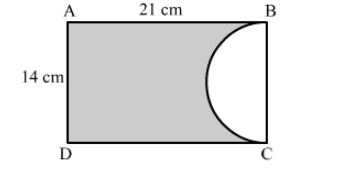# In the given figure, ABCD is a rectangle of dimensions 21 cm × 14 cm.`
Question:

In the given figure, ABCD is a rectangle of dimensions 21 cm × 14 cm. A semicircle is drawn with BC as diameter. Find the area and the perimeter of the shaded region in the figure.Solution:

= Area of the rectangle − Area of the semicircle

$=21 \times 14-\left\{\frac{1}{2} \times \pi \times\left(\frac{14}{2}\right)^{2}\right\}$

$=294-\left\{\frac{1}{2} \times \frac{22}{7} \times 7 \times 7\right\}$

$=294-77$

$=217 \mathrm{~cm}^{2}$

Therefore, area of shaded region is 217  cm2.

Length of the boundary (or perimeter) of the shaded region

$=\mathrm{AB}+\mathrm{AD}+\mathrm{DC}+\operatorname{arc} \mathrm{BC}$

$=\mathrm{AB}+\mathrm{AD}+\mathrm{DC}+\frac{1}{2} \times 2 \pi\left(\frac{14}{2}\right)$

$=21+14+21+\frac{22}{7} \times 7$

$=56+22$

$=78 \mathrm{~cm}$

Therefore, the perimeter of the shaded region is 78 cm.